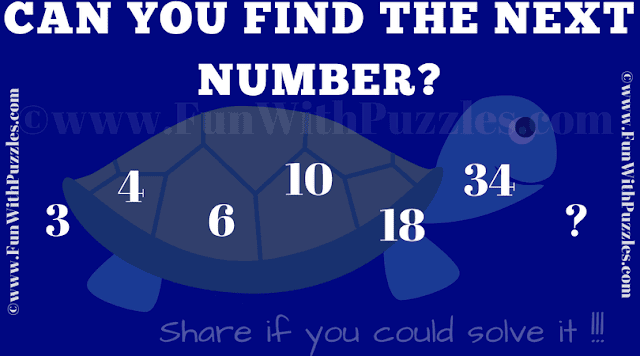This is Simple Puzzle in which one has to find the next number in the series. In the given Puzzle Picture, there are few numbers written in the sequence. These numbers follows some logical or Mathematical sequence. Your challenge is to decipher this sequence and tell the next missing number in the series. Can you tell what is the next number in this given series?Can you find the next number?

Answer of this "What is the Next Number? Simple Puzzle", can be viewed by clicking on button. Please do give your best try before looking at the answer.